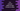# C++ program to convert miles to kilometers and kilometers to miles## How to convert miles to kilometers and kilometers to miles in C++:

In this post, we will learn how to convert miles to kilometers in C++ with examples. We will take the miles as input from the user, convert it to kilometers and print it back.

### Algorithm:

1 mile is equal to 1.60934 kilometers. So, if we multiply miles value by 1.60934, it will give us the conversion in kilometers.

We will keep the values in floating point variables.

• Read the number as input from the user
• Do the conversion
• Print it back

## C++ program to convert miles to kilometers:

Let’s take a look at the full program:

``````#include <iostream>

using namespace std;

int main()
{
float miles, km;

cout << "Enter the miles value: " << endl;
cin >> miles;

km = miles * 1.60934;

cout << miles << " miles = " << km << " km" << endl;

return 0;
}``````

Here,

• miles and km are two float values to store the miles and kilometers.
• It is asking the user to enter the miles value and reads that value to the miles variable.
• It finds the kilometer value by multiplying miles with 1.60934 and stores that value in km.
• The last line is printing both of these values.

If you run this program, it will print output as like below:

``````Enter the miles value:
30
30 miles = 48.2802 km

Enter the miles value:
22
22 miles = 35.4055 km``````

## C++ program to convert miles to kilometers by using a separate function:

We can also solve this program by using a separate function. We can create a function that will take the value of miles as the parameter and return the value in kilometers.

For example:

``````#include <iostream>

using namespace std;

float milesToKm(float miles){
return miles * 1.60934;
}

int main()
{
float miles, km;

cout << "Enter the miles value: " << endl;
cin >> miles;

km = milesToKm(miles);

cout << miles << " miles = " << km << " km" << endl;

return 0;
}``````

Here,

• We have created a new function milesToKm that takes the miles value as the argument and returns the kilometer value.
• Instead of calculating the value directly, we are calling milesToKm method to find the result.

If you run this program, it will give similar result. Using a separate function has its own advantage because you can call it from different places. For example, you can call this function from different files. If you make any change to this function, it will reflect on all other places.

## C++ program to convert kilometer to miles:

This is the reverse of what we did above. To convert a kilometer value to miles, we have to divide the kilometer value by 1.60934. That’s it.

Similar to the above program, we can take the kilometer value as input from the user, convert it to miles and print it back.

For example:

``````#include <iostream>

using namespace std;

float kmToMiles(float km){
return km/1.60934;
}

int main()
{
float miles, km;

cout << "Enter the kilometer value: " << endl;
cin >> km;

miles = kmToMiles(km);

cout << km << " km = " << miles << " miles" << endl;

return 0;
}``````

We are doing the reverse process here. If you run this program, it will give output as like below:

``````Enter the kilometer value:
35.4055
35.4055 km = 22 miles``````